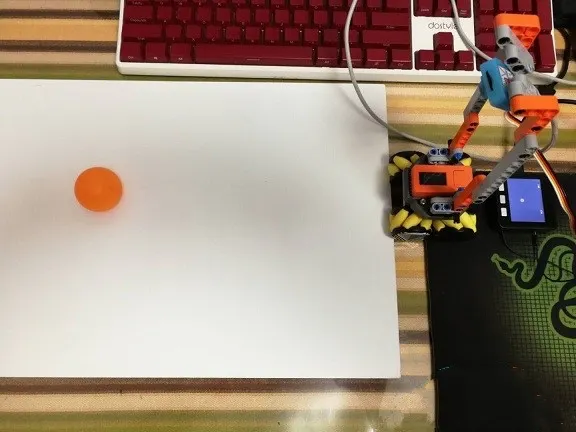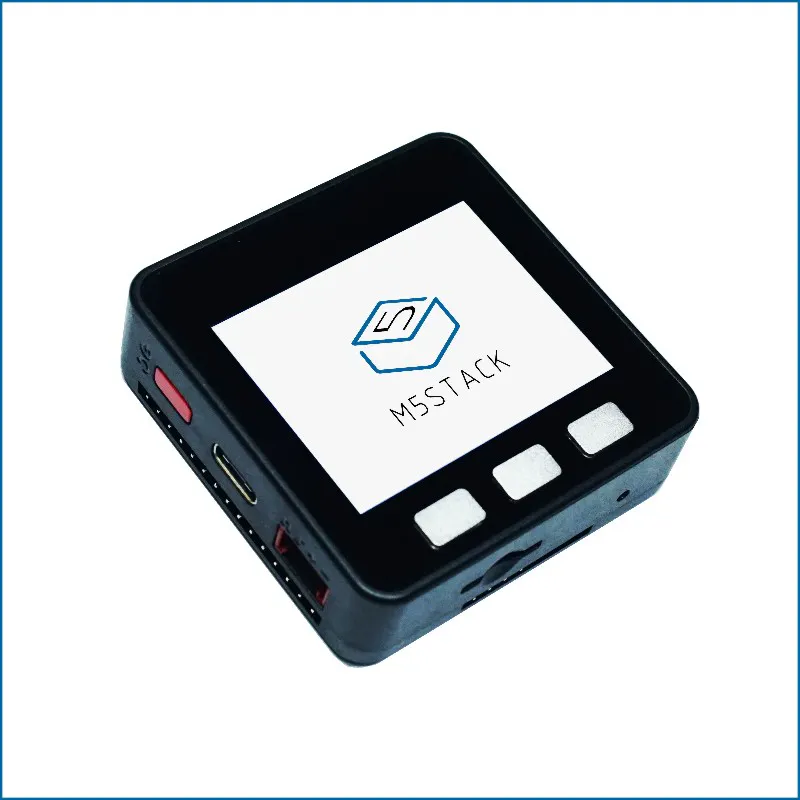Published © MIT

# UnitV with M5Stack Deep Learning and Object Detection

Thank the original author @Canghai

IntermediateFull instructions provided2 hours925## Things used in this project

### Hardware components

 M5Stack Unit-V
×1M5Stack ESP32 Basic Core IoT Development Kit
×1

### Software apps and online services

 Maixpy
 M5Stack UIFlow

Read more

## Code

### core

Python
```from m5stack import *
from m5ui import *
from uiflow import *
import json

setScreenColor(0x222222)

#rectangle0 = M5Rect(40, 5, 200, 170, 0xFFFFFF, 0xFFFFFF)
label1 = M5TextBox(39, 10, "Text", lcd.FONT_Default,0xFFFFFF, rotate=0)
label2 = M5TextBox(231, 12, "Text", lcd.FONT_Default,0xFFFFFF, rotate=0)
circle0 = M5Circle(81, 144, 10, 0xfdd708, 0xFFFFFF)

uart = None
dict2 = None
data1 = None
atom_x = None
atom_y = None

uart = machine.UART(1, tx=17, rx=16)
uart.init(115200, bits=8, parity=None, stop=1)
while True:

if uart.any():
dict2 = uart.readline()
data1 = json.loads(dict2)
atom_x=data1['ball_x']
atom_y=data1['ball_y']
label1.setText(str(atom_x))
label2.setText(str(atom_y))
circle0.setPosition((320-atom_x), (atom_y+20))
```

### unitV

Python
```#======2020-03-08 unitvuart
import image
#import lcd
import sensor
import sys
import time
import KPU as kpu
from fpioa_manager import *
import math
import KPU as kpu
from Maix import GPIO
import utime

#===========uart init
fm.register(34,fm.fpioa.UART1_TX)
fm.register(35,fm.fpioa.UART1_RX)
uart_out = UART(UART.UART1, 115200, 8, None, 1, timeout=1000, read_buf_len=4096)

#
task=kpu.load(0x00300000)
#1
labels=["1"] #You can check the numbers here to real names.
anchor = (0.33340788 * 16, 0.70065861 * 16, 0.18124964 * 16,0.38986752 * 16, 0.08497349 * 16,0.1527057 * 16)
a = kpu.init_yolo2(task, 0.7, 0.5, 3, anchor)
#
#v-train0.2,0.05
#

print("Load Done.")
sensor.reset()
sensor.set_pixformat(sensor.RGB565)
sensor.set_framesize(sensor.QVGA)
sensor.set_windowing((320, 224))
sensor.run(1)

print("Init Done.")

counter = 1 #
code_stake = [] #

ball_x = 0 #ballx
ball_y = 0 #bally

while(True):
img = sensor.snapshot()
code = kpu.run_yolo2(task, img)

if code:
if counter < 3: #3code_stake[]
code_stake = code_stake + code
counter = counter  + 1
else:
counter = 0
ball_x = 0
ball_y = 0
#i.rect()   i.rect()    i.rect()    i.rect()   x,y,w,h
for i in code_stake:
ball_x=i.x()
#ball_x=i.rect()
ball_y=i.y()
#ball_y=i.rect()

if counter == 0: #3
#uart
#==== send json str for ball_x and ball_y   round()
#s_json="{\"ball_x\":\""+str(round(ball_x))+"\",\"ball_y\":\""+str(round(ball_y))+"\"}"
s_json="{\"ball_x\":"+str(round(ball_x))+",\"ball_y\":"+str(round(ball_y))+"}"
uart_out.write(s_json+"\r\n")
print(s_json)
code_stake = [] #
utime.sleep_ms(50)
for i in code: #
img.draw_rectangle(i.rect())

else:
#lcd.display(img)
pass
a = kpu.deinit(task)
```

CangHai